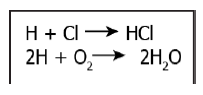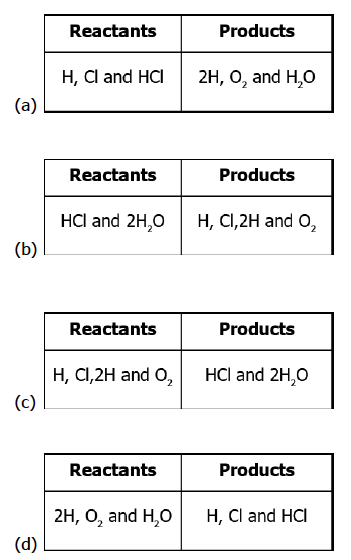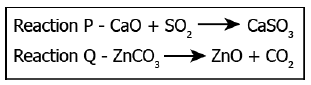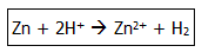# CBSE Class 10 Science MCQ Chapter 1 Chemical Reactions and Equations

## Chemical Reaction and Equation Class 10 MCQ

Chemical Reaction and Equation Class 10 MCQ are provided here to help students get updated with the new types of questions and their difficulty level. Class 10 Science Chapter 1 MCQ have become an important part of the exam. With the change in the exam pattern, the board had introduced MCQs in the Class 10 Science paper. So, to help students understand the different types of multiple choice questions, we have compiled the CBSE Class 10 Science MCQ Chapter 1 Chemical Reactions and Equations. It will help them to understand how MCQs are framed in the exam. So, accordingly students can practice the MCQ questions for Class 10 Science Chapter 1. They can also find the answers to Class 10 Chemistry Chapter 1 MCQs on this page.

Students can download these Chemical Reaction and Equation Class 10 MCQ in pdf format from the link below and refer to them anytime during their studies. We have also compiled the CBSE Class 10 Science MCQs for all chapters at one place to help students prepare better for the exams. Practising Class 10 Science Chapter 1 MCQ will boost students confidence level.

It is expected that a few of these Chemical Reaction and Equation Class 10 MCQs might be asked in the exam. So, students are advised to practise Class 10 Science Chapter 1 MCQ thoroughly. We have also provided the answers to CBSE Class 10 Science MCQ Chapter 1 Chemical Reactions and Equations, so that students can easily check their answers and evaluate their preparation level. These Chemical Reaction and Equation MCQ will give students an idea on new types of questions that can be framed in the exam from this chapter. To practise more questions on Class 10 Chemistry Chapter 1 MCQs, students can also solve the CBSE Class 10 Science Sample Papers based on the latest design and exam pattern. Below are the 20 MCQ Questions for Class 10 Science Chemistry Chapter 1 along answers.

## CBSE Class 10 Science MCQ Chapter 1 Chemical Reactions and Equations with Answers

Q1) Sodium and chlorine are reacted and as a result, sodium chloride is formed which is also called table salt. What option gives the reactants and products of the reaction?

(a) reactants – sodium; products – chlorine

(b) reactants – sodium and table salt; products – chlorine

(c) reactants – tables salt; products – sodium and chlorine

(d) reactants – sodium and chlorine; products – sodium chloride

Q2) Which of the following reactions can also be termed as thermal decomposition reaction?

(a) Combination Reaction

(b) Decomposition Reaction

(c) Displacement reaction

(d) Double displacement reaction

Q 3) The image shows some chemical reactions.Which option identifies the reactants and products of the reactions?Q4) A student performs an experiment to form aluminium chloride from aluminium and chlorine. Which options give the chemical equation of the reaction?

(a) Al + Cl2 → AlCl2

(b) 2Al + Cl2 → 2AlCl

(c) 2Al + 3Cl2 → 2AlCl3

(d) 3Al + 3Cl2 → 3AlCl3

Q5. Give the ratio in which hydrogen and oxygen are present in water by volume.

(a) 1:2

(b) 1:1

(c) 2:1

(d) 1:8

Q6) A researcher adds barium hydroxide to hydrochloric acid to form a white-colored barium chloride. Which option gives the balanced chemical equation of the reaction?

(a) HCl + Ba(OH)2 → BaCl2 + 2HOH

(b) 2HCl + Ba(OH)2 → BaCl2 + 2HOH

(c) 2HCl + Ba(OH)2 → BaH2 + 2HCl + O2

(d) HCl + 2Ba(OH) → 2BaCl2 + 2HOH + O2

Q7. One of the following processes does not involve a chemical reaction, that is:

(a) Melting of candle wax when heated

(b) Burning of candle wax when heated

(c) Digestion of food in your stomach

(d) Ripening of banana

Q8) A student writes a chemical equation of the reaction between carbon monoxide and hydrogen.

CO2 + 2H2 → CH3OH

How can the reaction be classified?

(a) The reaction is an example of a combination reaction as a compound separates into two compounds.

(b) The reaction is an example of a decomposition reaction as a compound dissociates into two compounds.

(c) The reaction is an example of a combination reaction as two compounds react to form a single compound.

(d) The reaction is an example of a decomposition reaction as two compounds react to form a single compound.

Q9) The chemical formula of magnesium oxide is?

(a) MgO2

(b) Mg2O

(c) MgO

(d) Mg(OH)2

Q10) A student learns that some products are formed as a result of combining two compounds while some compounds are formed as a result of dissociation of two compounds. The image shows two reactions.Which reaction is an example of a combination reaction and a decomposition reaction?

(a) both the reactions are examples of combination reaction

(b) both the reactions are examples of a decomposition reaction

(c) reaction P is an example of a combination reaction while reaction Q is an example of a decomposition reaction

(d) P is an example of a decomposition reaction while reaction Q is an example of a combination reaction

Q11) From the following which one is the example of chemical reaction?

(a) Grapes get fermented

(b) Breakdown of food

(c) Formation of curd

(d) All of the above

Correct Answer: Option (d) All of the above

Q12) A student adds lead and silver to two different test tubes containing an equal amount of copper sulphate solution. The student observes that the color of the solution in the test tube with lead changes. What explains the change in the colour of the solution?

(a) A displacement reaction takes place as lead replaces copper from the solution.

(b) A combination reaction takes place as lead combines with sulphate in the solution.

(c) decomposition reaction takes place as copper dissociates from sulphate in the solution.

(d) A double displacement reaction takes place as copper dissociates from sulphate and lead combines with sulphate in the solution.

Q13) Which of the reactions is used in black and white photography?

(a) Combination Reaction

(b) Decomposition Reaction

(c) Displacement reaction

(d) Oxidation reaction

Q14) What happens when lead nitrate reacts with potassium iodide?

(a) They will not react

(b) Large amount of hydrogen release

(c) Yellow ppt of lead iodide and potassium nitrate will be produced

(d) Evolution of gas will occur

Q15) The chemical reaction between potassium chloride and silver nitrate is given by the chemical equation.

AgNO3 + KCl → AgCl + KNO3

What can be inferred from the chemical equation?

(a) silver nitrate and potassium undergo a decomposition reaction to form silver chloride and potassium nitrate

(b) silver nitrate and potassium undergo a displacement reaction to form silver chloride and potassium nitrate

(c) silver nitrate and potassium undergo a combination reaction to form silver chloride and potassium nitrate

(d) silver nitrate and potassium undergo double displacement reaction to form silver chloride and potassium nitrate

Q16. Which of the following does show an oxidation reaction?

(a) Gain of oxygen

(b) Loss of oxygen

(c) Gain of hydrogen

(d) None of the above

Q17) The image shows a reaction between zinc and hydrogen.Which option shows oxidation?

(a) Zn → Zn+2

(b) 2H+ → H2

(c) Zn+2 → Zn

(d) H2 → 2H+

## Frequently Asked Questions on Chemical Reactions and Equations

### What is the chemical reaction for class 10th?

Chemical reactions are chemical changes in which reactants transform into products by making or breaking bonds (or both) between different atoms.

### What is a chemical reaction and equation with an example?

Representation of a chemical reaction in terms of symbols and chemical formulae of the reactants and products is known as a chemical equation.
Fe (s) + S (s) → FeS (s)

### What are 10 examples of a chemical reaction?

Below are the examples of chemical reactions:

1. Rusting of iron
2. Burning of candle
3. Burning of wood
4. Formation of curd from milk
5. Waste decomposition
6. Cooking food
7. Formation of caramel from sugar by heating
8. Baking of cookies and cakes.
9. Photosynthesis process
10. Digestion of food

We hope students have found this information on “CBSE Class 10 Science Chapter 1 Chemical Reaction and Equation MCQ” useful for their exam preparation. Keep learning and stay tuned for further updates on the CBSE Exam. Download BYJU’S App and subscribe to the YouTube channel to access interactive study videos.